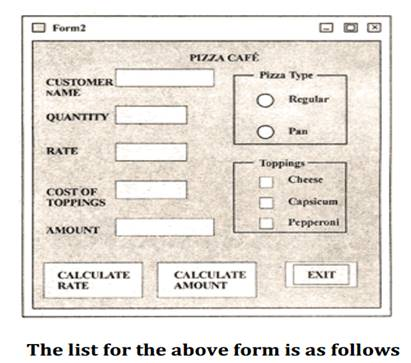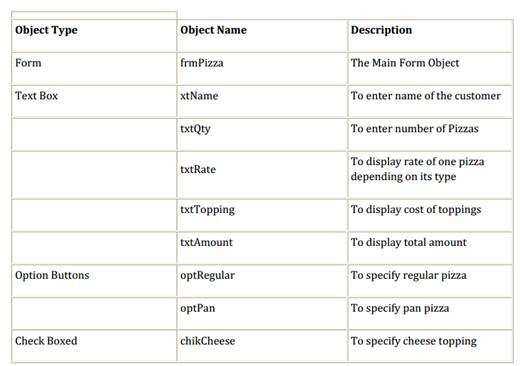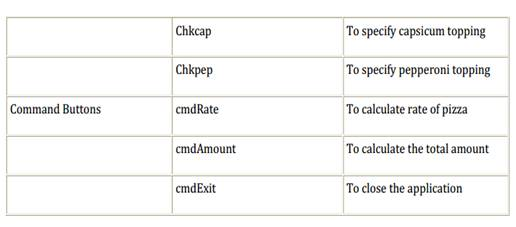1. /
2. CBSE
3. /
4. Class 12
5. /
6. Informatics Practices
7. /
8. CBSE Question Paper 2006...

# CBSE Question Paper 2006 class 12 Informatics Practices### myCBSEguide App

Download the app to get CBSE Sample Papers 2023-24, NCERT Solutions (Revised), Most Important Questions, Previous Year Question Bank, Mock Tests, and Detailed Notes.

CBSE Question Paper 2006 class 12 Informatics Practices conducted by Central Board of Secondary Education, New Delhi in the month of March 2006. CBSE previous year question papers with solution are available in myCBSEguide mobile app and cbse guide website. The Best CBSE App for students and teachers is myCBSEguide which provides complete study material and practice papers to cbse schools in India and abroad.

CBSE Question Paper 2006 class 12 Informatics Practices

## CBSE Question Paper 2006 class 12 Informatics Practices

### General Instructions:

• This question paper is divided into 3 sections.
• Section — A consists of 30 marks.
• Section — B and Section — C are of 20 marks each.

### Q1.  Answer the following questions:

i. Explain the term Metadata using suitable example. (2)
ii. What is a Financial Accounting system? Name any two entities or tables that could be a part of this system. (2)
iii. What is feasibility study with reference to SDLC? Explain. (2)
iv. Explain the concept of object-oriented modeling. (2)
v. Define Data Warehousing using suitable example. (2)

### Q2.  Answer the following questions:

i. What do you understand by the term Event-Driven Programming? Explain. (2)
ii. Write the names of any two properties and any two methods, which are common to the
checkbox object and option button object. (2)
iii. Differentiate between the Drive List Box and Dir List Box. Also name the most important
property of each of these controls, which needs to be set by the user. (2)
iv. What is the purpose of the Common Dialog control? List any two methods of the Common
Dialog Control. (2)
v. What do you understand by the term data-bound controls? Name any two data-bound
controls that can be used on a form. (2)

### Q3. Answer the following questions:

i. What do you understand by the term Equi – Join? Give an example. (2)
ii. Explain the difference between the SUBSTR and INSTR function of SQL with the help of an
example. (2)
iii. What are group functions of SQL? Name any two group functions. (2)
iv. Differentiate between the COMMIT and ROLLBACK commands of SQL.  (2)
v. Explain the usage of the IN OUT parameter of a PL/SQL procedure with the help of an
example. (2)

SECTION – B

### Q. 4. Read the following case study and answer the questions that follow:

The Pizza Cafe has computerized its billing. The following is the data entry screen used at their
outlet. The outlet offers two different types of pizzas, regular and pan pizzas. The price of a regular pizza is Rs. 90 and that of a pan pizza Rs. 110. The user can choose to have three different types of extra toppings if he wants. Each extra toppings costs Rs. 20.i. Write the commands to disable the textboxes txtKate, txt’l’opping, txt Amount and cmd Amount. (2)
ii. Write the code for cmd Rate to calculate the rate of the pizza and display it in txt Rate depending on the type of pizza selected by the customer. It should also enable the cmd Amount button. (4)
iii. Write the code for cmd Amount to calculate the total amount and display it in txt Amount. The total amount is calculated by first finding the cost of extra toppings selected by the customer. Remember that each extra topping costs Rs. 20 therefore if the user selects 2 toppings the cost of the toppings will be Rs. 40. Then add it to the rate and multiply the resultant amount by the quantity ordered.  (4)

### Q. 5. Answer the following questions:

i. Find the errors from the following code segment and rewrite the corrected code underlining
the correction made.  Dim num=10, las Integer
I = I
Do While 1 < 5
1 = 1 + 2
If num > 15
num = 0
Else
num = num — 3
Endlf
End While

ii. Give output of the following statements:  (a) INSTR (LTRIM (“INTERNATIONAL”), “na”)
(b) INT (4 – 7 * 3/2 + 5)

iii. Change the following code using FOR loop without effecting the output  Dim count, and
ans = 1
count = 20
DO
ans = ans * count
count = count -2
LOOP UNTIL count < = 10
Print ans

iv. Write a Visual Basic procedure which takes a number as argument and dis plays whether it is prime or not. A prime number is one, which is not divisible by any number other than by 1 and by itself. 

SECTION – C

i. Write the output produced by the following part of code in PL/SQL: (2)
DECLARE
A NUMBER: = 10;
B NUMBER: = 2;
BEGIN
WHILE B < 12 LOOP
IF A > B THEN
DBMS_OUTPUT.PUT_LINE (TO_CHAR(A));
ELSE
DBMS_OUTPUT.PUT_LINE(TO_CHAR(B));
END IF;
A : = A — 4;
B : = B + 3;
END LOOP;
END;

ii. Find the errors from the following PL/SQL code and rewrite the corrected code underlining
CREATE AS WELL AS REPLACE TRIGGER DEPT_UP
AFTER UPDATE ON DEPT FOR_EVERY ROW
DECLARE
v_num NUMBER (3);
BEGIN
SELECT COUNT (*) INTO v_num FROM Emp WHERE Dept ‘1O1’;
IF v_num > 5
Raise_application_error (—20001, ‘Cannot exceed 5’);
END;

iii. What do you understand by % ROWCOUNT with reference to cursors? What will happen if
you use the %ROWCOUNT attribute of a cursor that is not open? (2)

iv. Write a PL/SQL procedure called FACTORIAL that takes an integer as parameter, finds its
factorial and display it. (Factorial of a number is the product of all whole numbers less than
and equal to that number. For example, factorial of 4 = 4 x 3 x 2 x 1 = 24).  (4)

### Q. 7. Answer the questions base on the table CLUB given below:

Table: CLUB

 Column Name Data Type Size Constraint Description Member_No NUMBER 4 PRIMARY KEY Member Number Member_name VARCHAR2 30 NOT NULL Name of the member Address VARCHAR2 30 Address of the member Age NUMBER 3 > = 18 Age of the member Type VARCHAR2 10 Type of membership Fees NUMBER 6,2 Membership fees

i. Write the SQL command to create the table Club including the constraints. (2)
ii. Write the SQL command to display the details of the all the members whose type is
“Permanent” and fees is more than Rs. 1000. (2)
iii. Write a Pl/SQL code to increase the fees by 5% for a member number accepted from the use if the type of the membership is “Temporary”. (3)
iv. Write Pl/SQL code using and explicit cursor to display the details of all the “permanent”
members whose age is greater than 50. The code should also display the average membership fees of all such members. (3)

## CBSE Question Paper 2017 class 12 Informatics Practices

Download class 12 Informatics Practices question paper with solution from best CBSE App the myCBSEguide. CBSE class 12 Informatics Practices question paper 2017 in PDF format with solution will help you to understand the latest question paper pattern and marking scheme of the CBSE board examination. You will get to know the difficulty level of the question paper.

## Previous Year Question Paper for class 12 in PDF

CBSE question papers 2018, 2017, 2016, 2015, 2014, 2013, 2012, 2011, 2010, 2009, 2008, 2007, 2006, 2005 and so on for all the subjects are available under this download link. Practicing real question paper certainly helps students to get confidence and improve performance in weak areas.

To download CBSE Question Paper class 12 Accountancy, Chemistry, Physics, History, Political Science, Economics, Geography, Computer Science, Home Science, Accountancy, Business Studies and Home Science; do check myCBSEguide app or website. myCBSEguide provides sample papers with solution, test papers for chapter-wise practice, NCERT solutions, NCERT Exemplar solutions, quick revision notes for ready reference, CBSE guess papers and CBSE important question papers. Sample Paper all are made available through the best app for CBSE students and myCBSEguide website.### Test Generator

Create question paper PDF and online tests with your own name & logo in minutes.### myCBSEguide

Question Bank, Mock Tests, Exam Papers, NCERT Solutions, Sample Papers, Notes